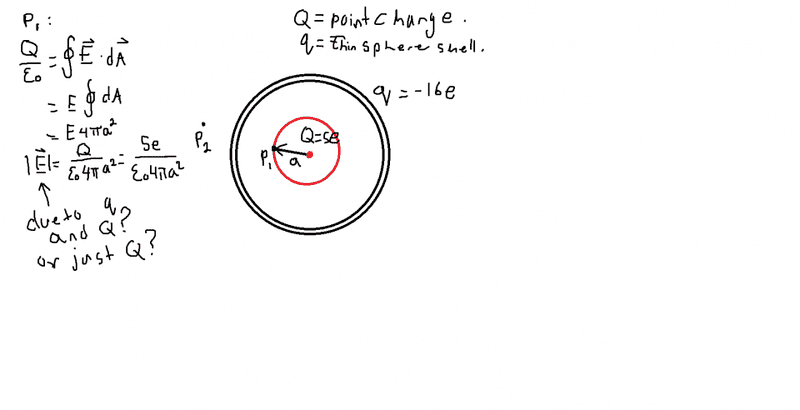# Gauss's Law and Electric Field Question.

sanhuy

## Homework Statement

figure shows, in cross section, a plastic, spherical shell
with uniform charge q=16e and radius R. A particle
with charge Q = 5e is at the center.What is the electric field
(magnitude and direction) at (a) point P1 at radial distance a from Q

My question is at the point P1 is the electric field that the book found using gauss's law the total electric field or just the electric field due to Q (the point charge only).

This is worked out example 23.03 in the book "fundementals of physics 10e" by halliday and resnick.
Thanks.

Q/ε = ∫Eda

## The Attempt at a Solution[/B]

Last edited:

Homework Helper

## Homework Statement

figure shows, in cross section, a plastic, spherical shell
with uniform charge q=16e and radius R. A particle
with charge Q = 5e is at the center.What is the electric field
(magnitude and direction) at (a) point P1 at radial distance a from Q

My question is at the point P1 is the magnitude of the electric field found using guass's law due to Q, or both Q and q?

What does Gauss' Law state?

sanhuy
What does Gauss' Law state?
I believe it states that the total electric flux out of / into a closed surface is equal to the charge enclosed divided by the permittivity. it pretty much relates the electric field at points on a closed surface to the
net charge enclosed by that surface.

sanhuy
Sorry, Let me rephrase my question because there may be some misinterpretation. is the electric field that the book found using gauss's law the total electric field or just the electric field due to Q (the point charge only).

Staff Emeritus
Homework Helper
Gold Member
2021 Award
Are you familiar with the shell theorem?

sanhuy
Are you familiar with the shell theorem?
No, not really. :\

sanhuy
wait, is the electric field inside of a shell zero due to symmetry if the charge is uniformly distributed on the spherical shell?

Homework Helper
Sorry, Let me rephrase my question because there may be some misinterpretation. is the electric field that the book found using gauss's law the total electric field or just the electric field due to Q (the point charge only).
E is the electric field at the points of the Gaussian surface (the red sphere in your figure).
What charge is enclosed by your red Gaussian sphere that goes through P1? Is q inside it?

•sanhuy
sanhuy
E is the electric field at the points of the Gaussian surface (the red sphere in your figure).
What charge is enclosed by your red Gaussian sphere that goes through P1? Is q inside it?

Well it only encloses the charge Q not q. So is the electric field calculated at P1 only due to Q? and the electric field due to q is zero at point P1 because of gauss's law?

is this correct?
thanks.

Staff Emeritus
Homework Helper
Gold Member
2021 Award
Well it only encloses the charge Q not q. So is the electric field calculated at P1 only due to Q? and the electric field due to q is zero at point P1 because of gauss's law?

is this correct?
thanks.
Right.

•sanhuy
sanhuy
Thanks guys.